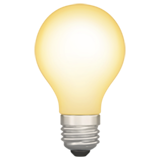Unit 1

# Classifications of Matter

Matter is typically characterized by:

1. Its physical state (gas, liquid, or solid)
2. Its composition (element, compound, or mixture)

## States of Matter

Gas — no fixed volume or shape, and can be compressed or expanded as there is space between the molecules.

Liquid — distinct volume but no distinct shape, cannot be compressed.

Solid — definite volume and shape, cannot be compressed.

## Composition

A pure substance (or substance) is matter that has distinct properties and a composition that does not vary sample to sample.

Two types of substances: elements and compounds.

• Elements are substances that cannot be decomposed into simpler substances.
• Compounds are substances composed of two or more elements (e.g. water).

Most elements can interact with other elements to form compounds. The elemental composition of a compound is always the same; this is called the law of constant composition.

In a mixture, each substance retains its chemical identity and properties. The composition of a mixture may vary in quantities.

Homogenous mixtures are also called solutions, even if they're not liquid.

# Properties of Matter

• Physical properties — can be observed without changing the identity and composition of the substance.
• Chemical properties — describe the way a substance may change to form other substances.
• For example, flammability.
• Intensive properties — do not depend on the amount of sample.
• These can be used to identify substances.
• Extensive properties — depend on the amount of sample.
• For example, mass and volume.

# Uncertainty in Measurement

## Definitions

• Exact numbers — numbers whose values are known exactly.
• Inexact numbers — numbers whose value have some uncertainty.
• All numbers obtained from measurement are inexact because of equipment or human errors.
• Precision — how closely individual measurements agree with one another.
• Accuracy — how closely individual measurements agree with the true value.

## Significant Figures

All digits of a measured quantity, including the uncertain (last) digit, are called significant figures.

Determine significant figures by reading a number left to right and counting digits, starting with the first non-zero digit.

The zeroes in a number that ends with zeroes but contains no decimal point are assumed to be not significant.

However, this can be more clearly expressed with scientific notation: 1.03 * 10^4 (three significant digits) 1.0300 * 10^4 (five significant digits)

## Significant Figures in CalculationsThe final answer should be reported with only one uncertain digit.

### Addition and Subtraction

The result has the same number of decimal places as the measurement with the fewest decimal places.

Example: 20.42 + 83.1 = 103.52 ⇒ 103.5

83.1 only has one decimal place, so the answer must be rounded to one decimal place.

### Multiplication and Division

The result contains the same number of significant figures as the measurement with the fewest significant figures.

Example: 6.221 * 5.2 = 32.3492 ⇒ 32

5.2 only has two significant figures, so the answer must be rounded to two significant figures.

# Dimensional Analysis

Dimensional analysis is the practice of multiplying or dividing units along with the numbers.

The key is using conversion factors, such as this one:

$\frac{2.54 \, \text{cm}}{1 \, \text{in.}}$

To calculate the units like this:

$(8.50 \, \text{in.}) * \frac{2.54 \, \text{cm}}{1 \, \text{in.}} = 21.6 \, \text{cm}$

The inches cancel out on top and bottom leaving just centimeters.